# Phasor Mathematics

## Mathematics for Electronics

• #### Question 1

Provide a definition for phasor, as the term applies to electrical calculations.

• #### Question 2

A series is a very important concept in mathematics. The common notation for a “series” looks something like this (a type of “geometric” series is shown here as an example):

$$\sum_{n=0}^\infty x^n$$

Explain what this notation means, and how we may approximate the value of a series for a finite value of n.

• #### Question 3

A common mathematical function known as factorial is represented by an exclamation point following a positive integer number. The following are all examples of factorials:

 1! = 1 2! = 2 3! = 6 4! = 24 5! = 120 6! = 720

Explain in your own words what this “factorial” function represents. What procedure (algorithm) would we use to arithmetically calculate the value of any factorial given to us?

• #### Question 4

Euler’s constant, that ubiquitous constant in mathematics symbolized by the letter e, may be found as the result of the following mathematical series:

$$e = \sum_{n=0}^\infty \frac{1}{n!}$$

Approximate the value of e in steps, using the following table:

 n n! 1 / n! ≈ e 0 1 2 3 4 5 6 7

Also, express this series as a partial sum up to n = 7.

• #### Question 5

The trigonometric function of cosine may be found as the result of an infinite series. Note that this series assumes the angle x to be expressed in units of radians, not degrees:

$$cos \ x = \sum_{n=0}^\infty (-1)^n\frac{x^{2n}}{(2n)!}$$

Approximate the cosine of 1 radian (cos1) in steps, using the following table, then write the partial sum expansion up to n = 5:

 n (−1)n x2n (2n)! ≈ cosx 0 1 2 3 4 5

• #### Question 6

The trigonometric function of sine may be found as the result of an infinite series. Note that this series assumes the angle x to be expressed in units of radians, not degrees:

$$sin \ x = \sum_{n=0}^\infty (-1)^n \ \frac{x^{2n+1}}{(2n + 1)!}$$

Approximate the sine of 1 radian (sin1) in steps, using the following table, then write the partial sum expansion up to n = 5:

 n (−1)n x2n 1 (2n 1)! ≈ sinx 0 1 2 3 4 5

• #### Question 7

Compare these three mathematical series, one for ex, one for cosx, and one for sinx:

$$e^x = 1+x+ \frac{x^2}{2!} + \frac{x^3}{3!} + \frac{x^4}{4!} + \frac{x^5}{5!} + + \frac{x^6}{6!} + + \frac{x^7}{7!} ...$$

$$cos \ x = 1 - \frac{x^2}{2!} + \frac{x^4}{4!} - \frac{x^6}{6!} + ...$$

$$sin \ x = x - \frac{x^3}{3!} + \frac{x^5}{5!} - \frac{x^7}{7!} + ...$$

Note similarities in the terms of these three series. Aside from signs, it would appear the cosine series contains all the even-powered terms of ex and the sine series contains all the odd-powered terms of ex. Leonhard Euler, the Swiss mathematician who lived from 1707 to 1783, found a connection between these three series which became known as Euler’s relation.

You too can find this same connection if you substitute jx for x in the first (ex) series, and then multiply all terms in the sine series by j. Leave the cosine series unaltered. Remember that j = √{−1} and that j2 = −1, j3 = −j, j4 = 1, j5 = j, etc.

• #### Question 8

A fascinating mathematical identity discovered by Leonhard Euler (1707-1783), regarded by some as the most beautiful equation in all of mathematics, relates five of mathematics’ fundamental constants together:

 ei π + 1 = 0

Use Euler’s relation to translate this identity into trigonometric terms, where the truth of this identity will become more evident.

• #### Question 9

If you have studied complex numbers, you know that the same complex quantity may be written in two different forms: rectangular and polar. Take for example the complex quantity [(√3)/2] j1/2. The following illustration shows this point located on the complex plane, along with its rectangular dimensions: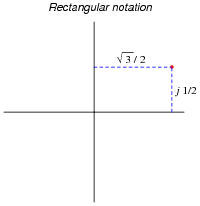Next, we see the same point, on the same complex plane, along with its polar coordinates: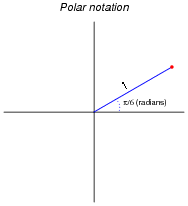Written out, we might express the equivalence of these two notations as such:

$$\frac{\sqrt{3}}{2} + j \frac{1}{2} = 1 \angle \frac{\pi}{6}$$

Expressed in a more general form, the equivalence between rectangular and polar notations would look like this:

 a + jb = c ∠Θ

However, a problem with the “angle” symbol (∠) is that we have no standardized way to deal with it mathematically. We would have to invent special rules to describe how to add, subtract, multiply, divide, differentiate, integrate, or otherwise manipulate complex quantities expressed using this symbol. A more profitable alternative to using the “angle” symbol is shown here:

 a + jb = c ejΘ

Explain why this equivalence is mathematically sound.

• #### Question 10

Electrical engineers usually express the frequency of an AC circuit in terms of angular velocity, measured in units of radians per second rather than cycles per second (Hertz, or Hz).

First, explain what a radian is. Next, write an equation relating frequency (f) in Hertz to angular velocity (ω) in radians per second. Hint: the relationship between the two is perhaps most easily understood in terms of a two-pole AC generator, or alternator, where each revolution of the rotor generates one full cycle of AC.

• #### Question 11

Suppose we have a simple two-pole AC generator, or alternator, the two stator windings on either side of the rotor connected together so as to function as a single winding: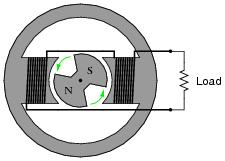Ideally, this machine will generate a sinusoidal output voltage as the rotor turns. Suppose now that we write index marks on the rotor shaft to measure its position as it turns, and we represent that position with the Greek letter “Theta” (Θ). It is purely arbitrary where we label the “zero” position on the shaft, so we choose to mark that point at some easily-identified point on the output waveform: the place where the coil output voltage peaks positive while rotating counter-clockwise. When spun, the instantaneous output voltage will then match the cosine function. In other words, the instantaneous output voltage will be proportional to the cosine of the shaft angle: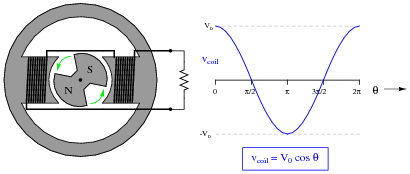We may represent the coil’s voltage with the equation vcoil = V0 cosΘ. If we precisely know the peak voltage value (V0) and the shaft position (Θ), we may precisely predict the coil voltage at any instant in time (vcoil).

However, there is a more complete way of describing what is happening in this alternator. The equation vcoil = V0 cosΘ is adequate for predicting coil voltage from shaft position, but it is not adequate for doing the reverse: predicting shaft position from coil voltage. Note that there are only two points on the voltage waveform where a voltage value corresponds to a unique shaft position, and those points are the positive and negative peaks. At any other value of instantaneous voltage (−V0

< vcoil < V

0), there are multiple possible shaft positions. The most obvious example of this is where vcoil = 0. Here, the shaft position could be [(π)/2] radians, or it could be [(3 π)/2] radians. Note that we consider the positive peak to occur at only one position (0 radians) and not two positions (0 and 2 π radians) in one revolution because 2 π radians is identical to 0 radians just as 360 degrees is equivalent to 0 degrees.

In order to uniquely describe the alternator’s shaft position in terms of output voltage, we need more information than just the instantaneous voltage at one coil. What we need is another coil, an imaginary coil, shifted in angular position from the first coil: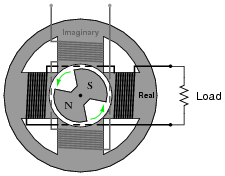Like the first coil (the real coil), the imaginary coil’s output voltage will also be sinusoidal. However, it will generate an output voltage at a different phase than the real coil’s output voltage.

Plot the waveform of the imaginary coil’s voltage, superimposed on the waveform of the real coil’s output voltage, and then write an equation expressing both instantaneous voltages as a complex sum: the real coil’s voltage as a real number and the imaginary coil’s voltage as an imaginary number (complete with the j prefix). Then, use Euler’s relation to re-write this complex sum as a complex exponential expression.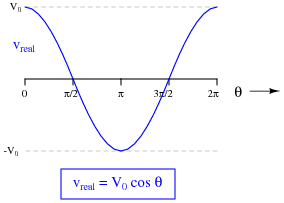• #### Question 12

Impedance is defined as the complex ratio of voltage and current:

 Z = V I

We may determine the complex definition of impedance provided by an inductor if we consider the equation relating inductor voltage and inductor current:

 v = L d dt [ i(t) ]

First, substitute a phasor expression of current into the above equation, then differentiate with respect to time to obtain an expression for voltage:

 i(t) = ej ωt

 v = L d dt [ ej ωt ]

Then, divide the two phasor expressions to obtain an expression for inductive impedance.

• #### Question 13

Impedance is defined as the complex ratio of voltage and current:

 Z = V I

We may determine the complex definition of impedance provided by an capacitor if we consider the equation relating capacitor voltage and capacitor current:

 i = C d dt [ v(t) ]

First, substitute a phasor expression of voltage into the above equation, then differentiate with respect to time to obtain an expression for current:

 v(t) = ej ωt

 i = C d dt [ ej ωt ]

Then, divide the two phasor expressions to obtain an expression for capacitive impedance.